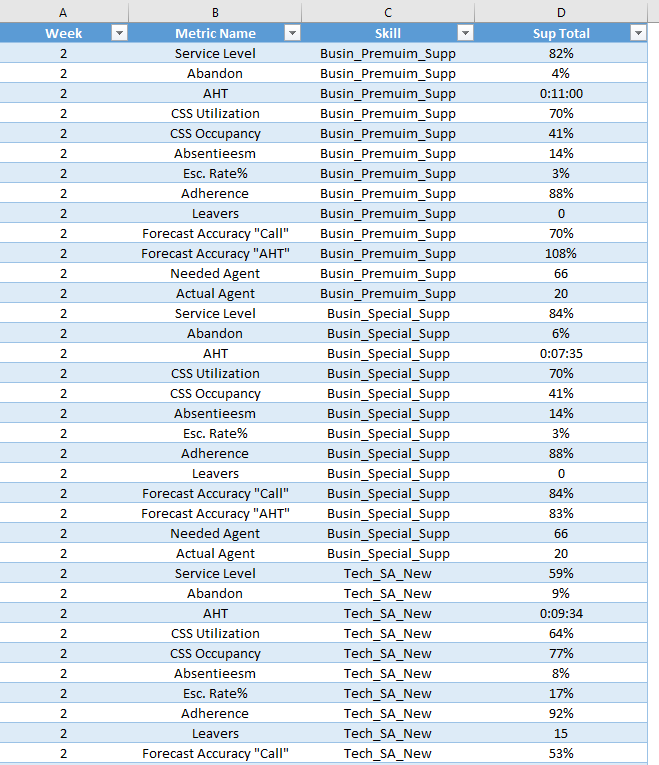cancel
Showing results for
Did you mean:Helper I

## data modeling with multiple format

Hello,

please, I need your useful ideas in how to preview a report which its value column have multiple formats. I ask that's the best data modeling to be able getting visual reports.

in recap: I ask if can I use the [Metric Name] column in  X-Axis then filter by [Week] or [Skill], or another preview that could show me the value by [Metric] or the value by [Skill]. or get a complete insight which could measure the [metric] by [Skill] and vs

The Data Modelthanks a lot,Microsoft

From my point of view, I don't think it is a good practice to put different formats data into a single column. So I would suggest you unify the formats for the column to Decimal Number type in this scenario. The percentage(80%) and integer(66) type data is easy to be changed to decimal number type. For the Time(0:11:00) type data, you can convert it to seconds or minutes first, then change it to decimal number.The formula below to create new calculate column to unify the formats is for your reference.

```Unified Column =
IF (
Data[Metric Name] = "AHT",
MINUTE ( Data[Sup Total] )
+ SECOND ( Data[Sup Total] ) / 60,
VALUE ( Data[Sup Total] )
)
```Regards